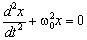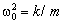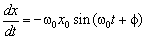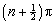# Physics Assignment Help With Simple Harmonic Motion

1. Home
2. Physics
3. Simple Harmonic Motion

## 7.1 Simple Harmonic Motion

Simple harmonic motion is important in practice because it is a good approximation to the free oscillations in many physical situations. Automobiles are usually suspended on springs, and an automobile would oscillate up and down in a way approximating SHM after the wheels hit a bump, unless steps were taken to stop it. Simple harmonic motion occurs when forces or torques that return an object to its equilibrium position are proportional to displacements from those positions. Many of the oscillatory motions in nature are SHM to a good approximation because, for a sufficiently small displacement of any body from equilibrium, the restoring force or restoring torque isproportional to the displacement.

Oscillating objects have energy and if energy is removed from the oscillations they die away. Pure SHM continues indefinitely when no energy is removed. Oscillations of real system are invariably damped to some extent and thus motions are never exactly SHM. Damping of oscillations is considered in next section.

Example of objects that oscillate with SHM to a very good approximation are provided by a mass on the end of a spring after the spring has been extended, a pendulum after it has been pulled sideways from its vertical position, a ball making small excursions back and forth inside a smooth upturned bowl, or a fixed mass of liquid oscillating up and down in a U-shaped tube. The variation with time of many physical observables other than spatial or angular displacements is also well described within the same formalism as SHM. An example is that of charge oscillating back and forth between the plates of a capacitor in a circuit with an inductor of small resistance. The equation representing simple harmonic motion is explained below… (ii)

with… (iii)

It can be verified by substitution that the solution to equation (ii) is

x(t) = x0cos (w0t + f)

where the constant x0 is the amplitude of the oscillations. The argument of the cosine term, w0t+f, is called the phase of the oscillation and the constant f is a phase angle. The parameter w0 is called the natural angular frequency of the oscillation and equation (i) was rewritten in the form of equation (ii) in order to exhibit clearly the relation between angular frequency, mass, and stiffness parameter k.

The solution of the second-order differential equation (ii) contains two constants as do the solutions to all such equations. These constants can be determined if the displacement and speed are both known at a given time. For example, if the mass is displaced by a certain amount x0 and released from rest at time zero, the initial conditions that determine the two constant are that at t = 0 the speed dx/dt is zero and the displacement x is x0. The speed isPutting this equation to zero at time zero gives the angle f equal to zero. The amplitude x0 is then identified as the initial displacement. When the displacement is a maximum, at values of w0t equal towith n any integer, the displacement is zero.

### Email Based Assignment Help in Simple Harmonic Motion

We are the leading online Assignment Help provider. Find answers to all of your doubts regarding the Simple Harmonic Motion. Assignmenthelp.net provides homework, Assignment Help to the school, college or university level students. Our expert online tutors are available to help you in Simple Harmonic Motion. Our service is focused on: time delivery, superior quality, creativity and originality.

To submit physics Simple Harmonic Motion assignment click

Following are some of the areas in physics Simple Harmonic Motion which we provide help:

Simple Harmonic Motion | Simple Harmonic Motion Problems | Physics Online Tutor | Physics Assignment Help | Physics Tutors | Physics Homework Help | Physics Tutor | Physics Help | Physics Projects | Physics Problems | Physics Online Help | Physics Online Tutoring | High School Physics | Homework Tutor | Physics Experiments | Online Tutoring